Test: Eigenvalues & Eigenvectors - 1

# Test: Eigenvalues & Eigenvectors - 1 - Mechanical Engineering

Test Description

## 20 Questions MCQ Test GATE Mechanical (ME) 2023 Mock Test Series - Test: Eigenvalues & Eigenvectors - 1

Test: Eigenvalues & Eigenvectors - 1 for Mechanical Engineering 2023 is part of GATE Mechanical (ME) 2023 Mock Test Series preparation. The Test: Eigenvalues & Eigenvectors - 1 questions and answers have been prepared according to the Mechanical Engineering exam syllabus.The Test: Eigenvalues & Eigenvectors - 1 MCQs are made for Mechanical Engineering 2023 Exam. Find important definitions, questions, notes, meanings, examples, exercises, MCQs and online tests for Test: Eigenvalues & Eigenvectors - 1 below.
Solutions of Test: Eigenvalues & Eigenvectors - 1 questions in English are available as part of our GATE Mechanical (ME) 2023 Mock Test Series for Mechanical Engineering & Test: Eigenvalues & Eigenvectors - 1 solutions in Hindi for GATE Mechanical (ME) 2023 Mock Test Series course. Download more important topics, notes, lectures and mock test series for Mechanical Engineering Exam by signing up for free. Attempt Test: Eigenvalues & Eigenvectors - 1 | 20 questions in 60 minutes | Mock test for Mechanical Engineering preparation | Free important questions MCQ to study GATE Mechanical (ME) 2023 Mock Test Series for Mechanical Engineering Exam | Download free PDF with solutions
 1 Crore+ students have signed up on EduRev. Have you?
Test: Eigenvalues & Eigenvectors - 1 - Question 1

### Find the sum of the Eigenvalues of the matrix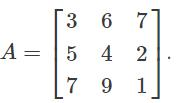Detailed Solution for Test: Eigenvalues & Eigenvectors - 1 - Question 1

According to the property of the Eigenvalues, the sum of the Eigenvalues of a matrix is its trace that is the sum of the elements of the principal diagonal.
Therefore, the sum of the Eigenvalues = 3 + 4 + 1 = 8.

Test: Eigenvalues & Eigenvectors - 1 - Question 2

### Find the Eigenvalues of matrix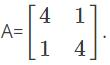Detailed Solution for Test: Eigenvalues & Eigenvectors - 1 - Question 2

A - λI = 0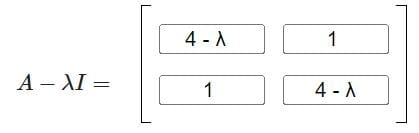Now, After taking the determinant: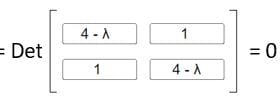(4 - λ)2 - 1 = 0

16 + λ2 - 8λ - 1 = 0

λ2 - 8λ + 15 = 0

- 3) (λ - 5) = 0

λ = 3, 5

Test: Eigenvalues & Eigenvectors - 1 - Question 3

### All the four entries of the 2 × 2 matrix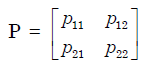are nonzero, and one of its eigen values is zero. Which of the following statements is true?

Detailed Solution for Test: Eigenvalues & Eigenvectors - 1 - Question 3

One eigen value is zero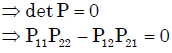Test: Eigenvalues & Eigenvectors - 1 - Question 4

The eigen values of the following matrix are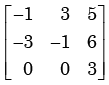Detailed Solution for Test: Eigenvalues & Eigenvectors - 1 - Question 4

Let the matrix be A.  We know, Trace (A) = sum of eigen values.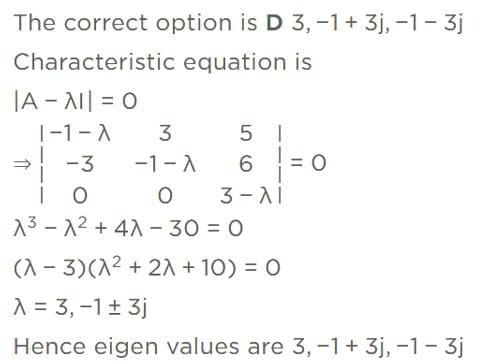Test: Eigenvalues & Eigenvectors - 1 - Question 5

The three characteristic roots of the following matrix A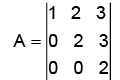Detailed Solution for Test: Eigenvalues & Eigenvectors - 1 - Question 5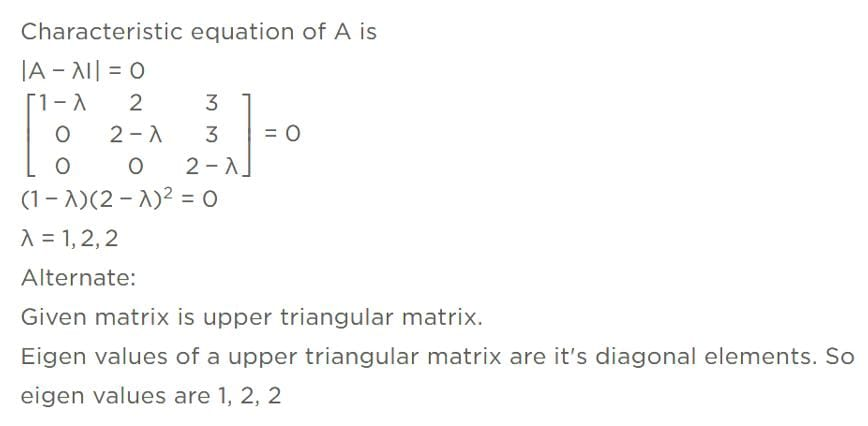Test: Eigenvalues & Eigenvectors - 1 - Question 6

The sum of the eigenvalues of the matrix given below is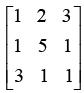Detailed Solution for Test: Eigenvalues & Eigenvectors - 1 - Question 6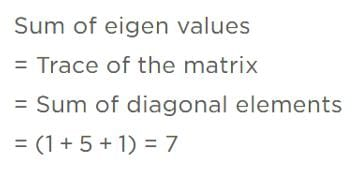Test: Eigenvalues & Eigenvectors - 1 - Question 7

For which value of x will the matrix given below become singular?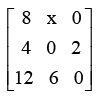Detailed Solution for Test: Eigenvalues & Eigenvectors - 1 - Question 7

Let the given matrix be A.  A is singular.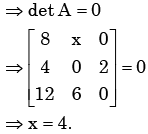Test: Eigenvalues & Eigenvectors - 1 - Question 8

Eigen values of a matrix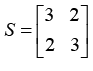are 5 and 1. What are the eigen values of the matrix S2  = SS?

Detailed Solution for Test: Eigenvalues & Eigenvectors - 1 - Question 8

We know If λ be the eigen value of A ⇒λ2 is an eigen value of A2 .

For S matrix, if eigen values are λ1, λ2, λ3,... then for S² matrix, the eigen values will be λ²1 λ²2 λ²3......
For S matrix, if eigen values are 1 and 5 then for S² matrix, the eigen values are 1 and 25

Test: Eigenvalues & Eigenvectors - 1 - Question 9

The number of linearly independent eigenvectors of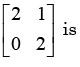Detailed Solution for Test: Eigenvalues & Eigenvectors - 1 - Question 9

Number of linear independent vectors is equal to the sum of Geometric Multiplicity of eigen values. Here only eigen value is 2.

To find Geometric multiplicity find n-r of (matrix-2I), where n is order and r is rank.

Rank of obtained matrix is 1 and n = 2 so n-r = 1. Therefore the no of linearly independent eigen vectors is 1

Test: Eigenvalues & Eigenvectors - 1 - Question 10

The eigenvectors of the matrix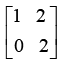are written in the form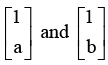. What is a + b?

Detailed Solution for Test: Eigenvalues & Eigenvectors - 1 - Question 10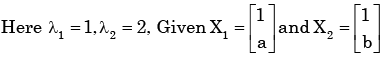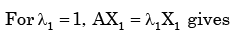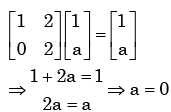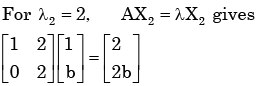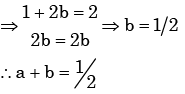Test: Eigenvalues & Eigenvectors - 1 - Question 11

One of the Eigenvectors of the matrix A =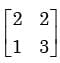is

Detailed Solution for Test: Eigenvalues & Eigenvectors - 1 - Question 11

The eigen vectors of A are given by  AX= λ X

So we can check by multiplication.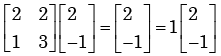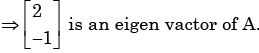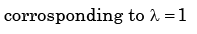Test: Eigenvalues & Eigenvectors - 1 - Question 12

The minimum and the maximum eigen values of the matrix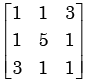are –2 and 6, respectively. What  is the other eigen value?

Detailed Solution for Test: Eigenvalues & Eigenvectors - 1 - Question 12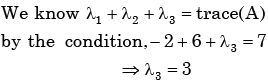Test: Eigenvalues & Eigenvectors - 1 - Question 13

The largest eigenvalue of the matrix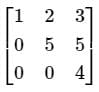is

Detailed Solution for Test: Eigenvalues & Eigenvectors - 1 - Question 13

Concept:

If A is any square matrix of order n, we can form the matrix [A – λI], where I is the nth order unit matrix.

The determinant of this matrix equated to zero

i.e. |A – λI| = 0 is called the characteristic equation of A.

The roots of the characteristic equation are called Eigenvalues or latent roots or characteristic roots of matrix A.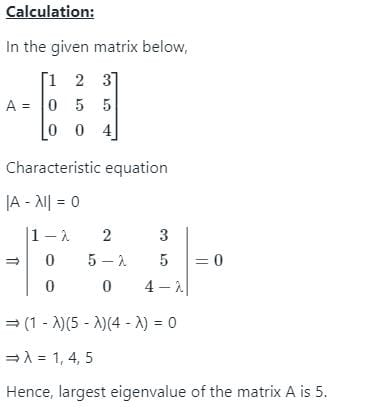Test: Eigenvalues & Eigenvectors - 1 - Question 14

The state variable description of a linear autonomous system is, X= AX,

Where X is the two dimensional state vector and A is the system matrix given by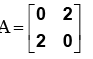The roots of the characteristic equation are

Detailed Solution for Test: Eigenvalues & Eigenvectors - 1 - Question 14

Characteristic equation will be :λ2 -4 =0 thus root of characteristic equation will be +2 and - 2.

Test: Eigenvalues & Eigenvectors - 1 - Question 15

For the matrix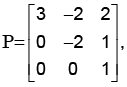s one of the eigen values is equal to -2. Which of the following  is an eigen vector?

Detailed Solution for Test: Eigenvalues & Eigenvectors - 1 - Question 15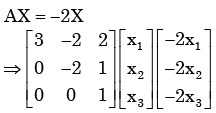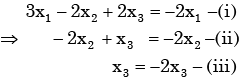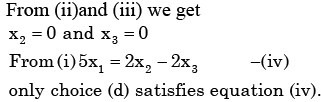Test: Eigenvalues & Eigenvectors - 1 - Question 16

x=[x1x2…..xn]T is an n-tuple nonzero vector. The n×n matrix V=xxT

Detailed Solution for Test: Eigenvalues & Eigenvectors - 1 - Question 16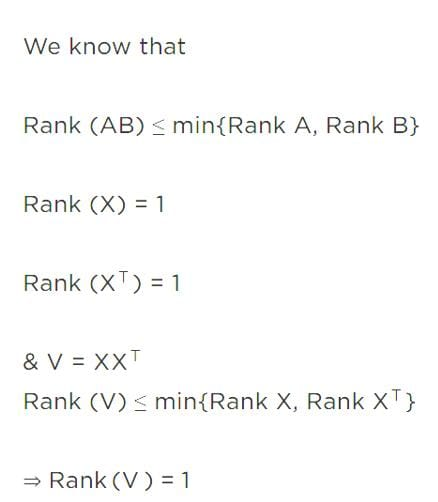Test: Eigenvalues & Eigenvectors - 1 - Question 17

Let A be an n × n complex matrix. Assume that A is self-adjoint and let B denotes the inverse of (A + iIn). Then all eigenvalues of (A - iIn)B are

Detailed Solution for Test: Eigenvalues & Eigenvectors - 1 - Question 17

Concept:

(i) A complex matrix A is said to be self-adjoint if A = A* where A* = A-T

(ii) Eigenvalues of a self-adjoint matrix are real

Explanation:

A is an n × n complex matrix and A is self-adjoint so

A = A*

B denotes the inverse of (A + iIn).

So B = (A + iIn)-1

Let λ be an eigenvalue of A

then λ + i is an eigenvalue of A + iIn

and (λ + i)-1 is an eigenvalue of B

Also λ - i is an eigenvalue of A - iIn

Hence eigenvalue of (A - iIn)B

= (λ - i)(λ + i)-1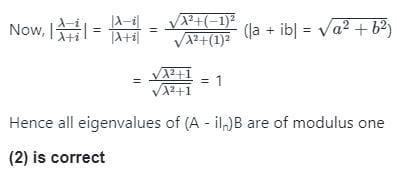Test: Eigenvalues & Eigenvectors - 1 - Question 18

If the rank of a (5×6) matrix Q is 4, then which one of the following statements is correct?

Detailed Solution for Test: Eigenvalues & Eigenvectors - 1 - Question 18

Rank of a matrix is equal to the No. of linearly independent row or no. of linearly  independent column vector.

Test: Eigenvalues & Eigenvectors - 1 - Question 19

The trace and determinate of a 2 ×2 matrix are known to be – 2 and – 35 respectively. Its eigenvalues are

Detailed Solution for Test: Eigenvalues & Eigenvectors - 1 - Question 19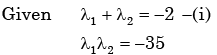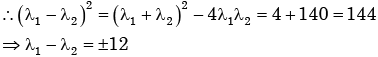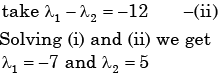Test: Eigenvalues & Eigenvectors - 1 - Question 20

Identify which one of the following is an eigenvector of the matrix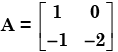Detailed Solution for Test: Eigenvalues & Eigenvectors - 1 - Question 20

Eigen Value (λ ) are 1,− 2.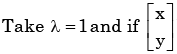be the eigen  of A. Corresponding

To λ then.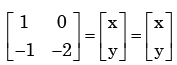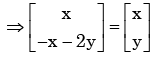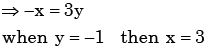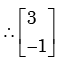be the eigen vector corrosponding to λ = 1

## GATE Mechanical (ME) 2023 Mock Test Series

27 docs|243 tests
Information about Test: Eigenvalues & Eigenvectors - 1 Page
In this test you can find the Exam questions for Test: Eigenvalues & Eigenvectors - 1 solved & explained in the simplest way possible. Besides giving Questions and answers for Test: Eigenvalues & Eigenvectors - 1, EduRev gives you an ample number of Online tests for practice

## GATE Mechanical (ME) 2023 Mock Test Series

27 docs|243 tests(Scan QR code)